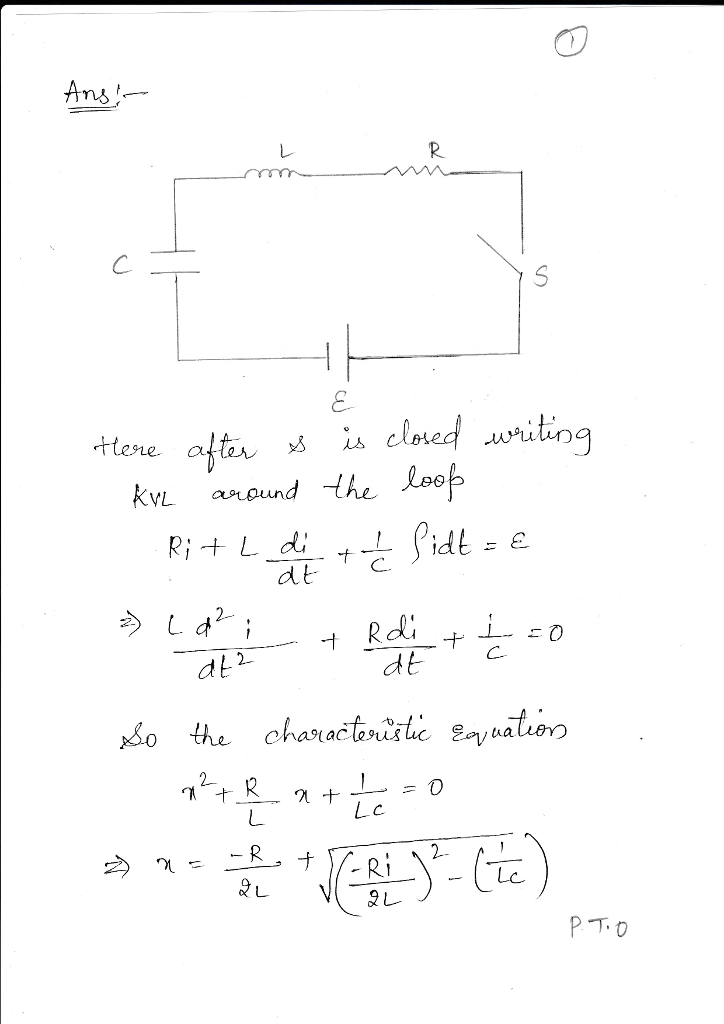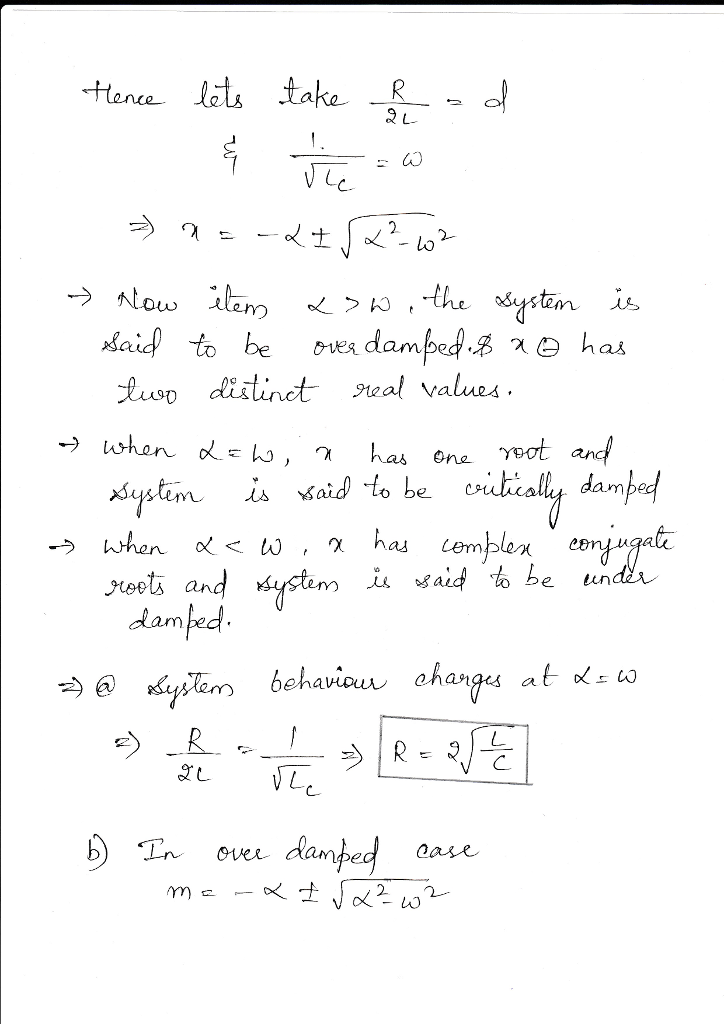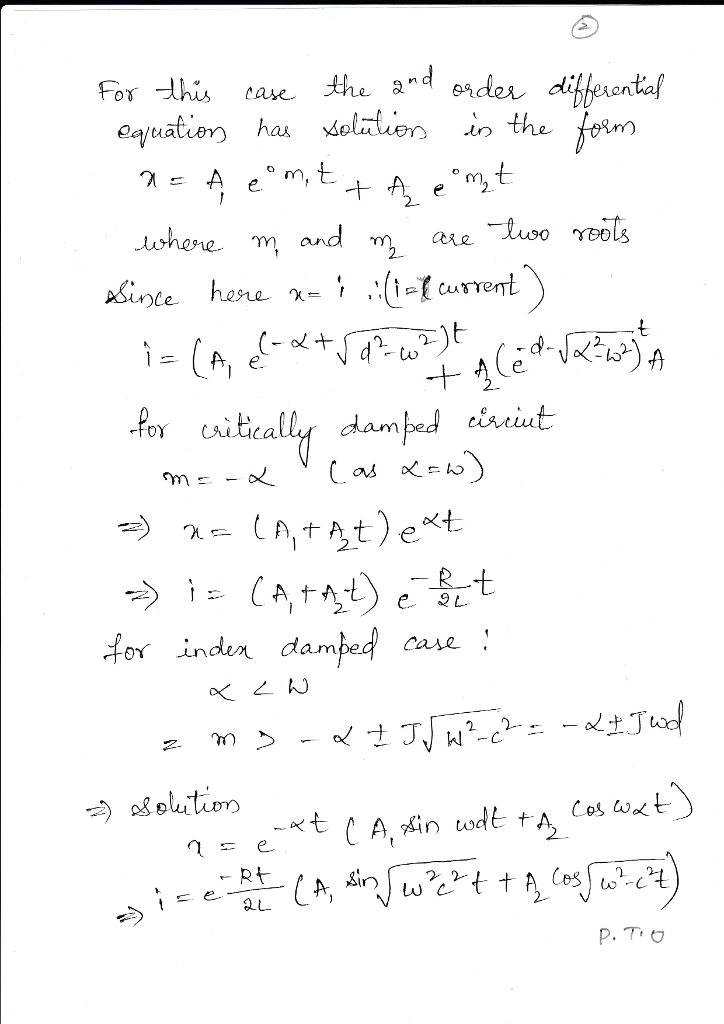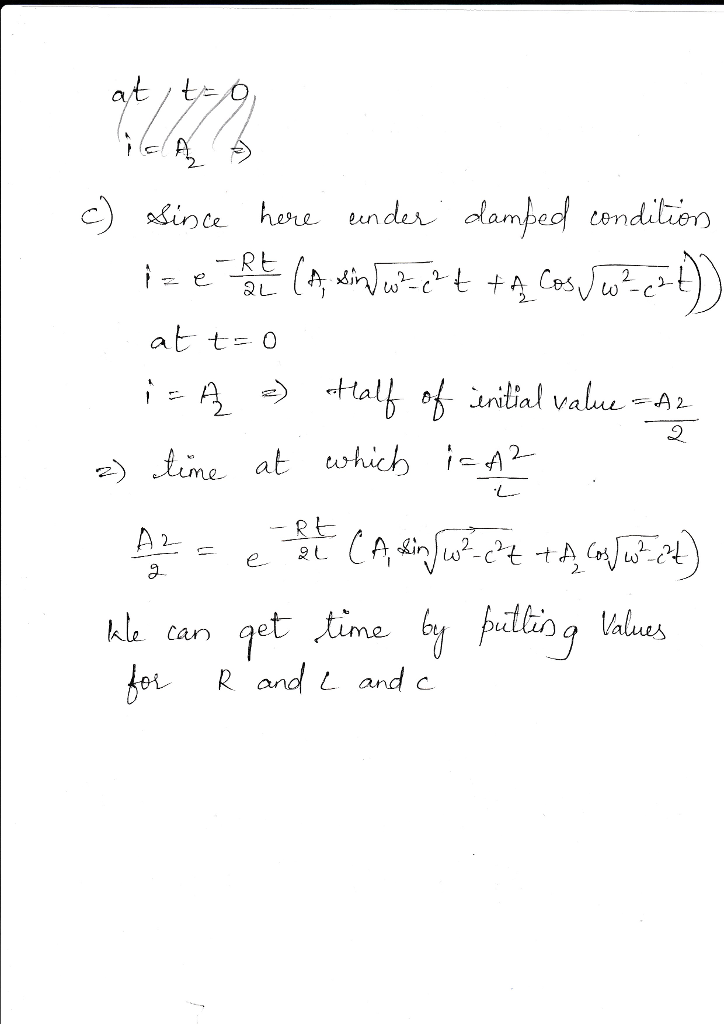# Homework Solution: A Resistor, R is connected in series to a capacitor, C, an inductor, L, a switch, S, and a battery supplying an EMF, epsilon. At time, t =…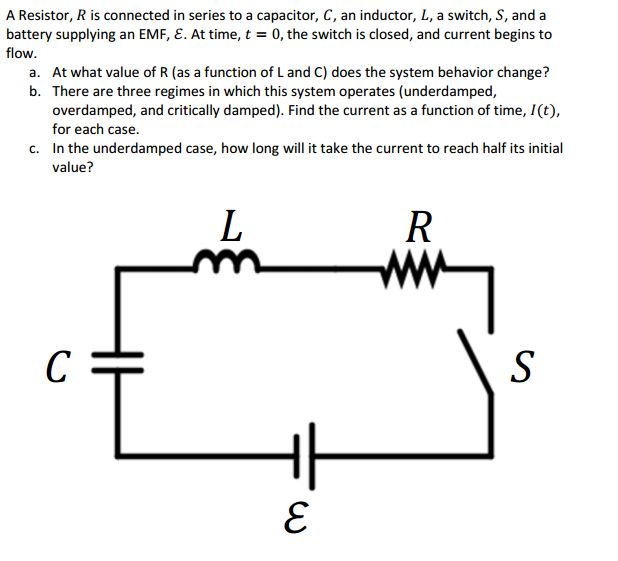A Resistor, R is connected in series to a capacitor, C, an inductor, L, a switch, S, and a battery supplying an EMF, epsilon. At time, t = 0, the switch is closed, and current begins to flow. a. At what value of R (as a function of L and C) does the system behavior change? b. There are three regimes in which this system operates (underdamped, overdamped, and critically damped). Find the current as a function of time, I(t), for each case. c. In the underdamped case, how long will it take the current to reach half its initial value?